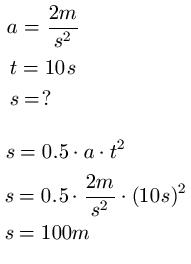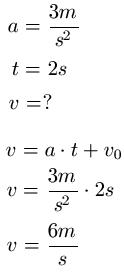# How does the steady speed change?

## Evenly accelerated movement

The uniformly accelerated motion is covered in this chapter. We will use examples to explain what this is all about. Of course, we will also show you the required formulas and provide exercises and solutions so that you can get fit with this area.In the last section, we covered uniform motion. As a reminder: This is a movement of an object that is always the same speed, i.e. it is neither faster nor slower. Before we get to accelerated motion, there are a few very basic math you should know. If, while reading the following section, you realize that you are having math difficulties, please read the articles at the links below. Everyone else can save this:

Show:

### The evenly accelerated movement

Now let's take care of the evenly accelerated movement. This has the following properties:

• The speed of the object changes, becomes either faster or slower
• Thus the acceleration - usually designated with "a" - is not equal to zero
• The acceleration is always constant in the case of a uniformly accelerated movement

So again: the acceleration is always the same. Example: a = 5m / s2. This means that the object is moving at 5m / s2 accelerated. "A" does not change during the movement, otherwise the acceleration would no longer be uniform.

Show:

### Calculate uniformly accelerated movement (formula)

There are three laws of uniformly accelerated motion. These laws provide information too Distance, acceleration, time, initial speed and initial distance.

Formula uniformly accelerated movement (distance-time law):

• s = 0.5 a t2 + vO · T + s0
• "s" is the distance in meters [m]
• "a" is the acceleration in meters per second square [m / s2]
• "t" is the time in seconds [s]
• "s0"is the starting path [m]

If the movement starts from a standstill and from the starting point, the formula simplifies to: s = 0.5 a t2

Formula uniformly accelerated movement (speed-time law):

• v = a t + v0
• "v" is the speed in meters per second [m / s]
• "a" is the acceleration in meters per second square [m / s2 ]
• "t" is the time in seconds [s]
• "v0"is the initial speed in meters per second [m / s]

If the movement begins without a start speed, the formula is simplified to: v = a t
Formula uniformly accelerated movement (acceleration-time law):

• a = constant
• "a" is the acceleration in meters per second square [m / s2]

### Evenly accelerated movement: examples

Time to count one or the other example of a steadily accelerated movement.

Example 1:Example 2:### Conversion of units

Many students have problems converting units. For this reason, we are going to give you a short summary of the most important conversion rules.

• 100cm = 1m = 0.001km
• 1m / s = 3.6km / h

Left:

### Who's Online

We have 184 guests online